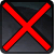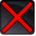# [passive]Conditions

Used by

## Related effects, buffs and debuffs

Please click on an effect below to view its details.

• [hidden] [does not expire]Effect #1

 Slot: Buff Duration: passive Tick rate: does not tick # occurrences: 0
• On Apply

Perform the following actions:

• Immobilize but allow rotation
• Grant Immunity
- Tag = (string)
- Ability Spec = (int) 16141047602584633006
- Damage Type List = Int(5)
- Damage Channel List = Int(1)
• Unknown (192)
• [hidden] [1s]Effect #2

 Slot: Buff Duration: 1s Tick rate: does not tick # occurrences: 0
• When effect expires

Perform the following actions:

• Add effect #3 to TARGET from TARGET
• Add effect #4 to TARGET from TARGET
• [hidden] [does not expire]Effect #3

 Slot: Buff Duration: passive Tick rate: 3s # occurrences: 0 Conditions: Can only by called by other effects
• When effect is applied
• When effect ticks

Only when the following conditions are met:

• If TARGET is not in combat

Perform the following actions:

• Execute Hydra Hook
- Event Name = (string) Agro_Placeholder
- Object Self = (int) 1
• [hidden] [does not expire]Effect #4

 Slot: Buff Duration: passive Tick rate: 2s # occurrences: 0 Conditions: Can only by called by other effects
• On Apply

Perform the following actions:

• Unknown (183)
- Unknown (303) = (bool) true
- Unknown (306) = (bool) true
- Unknown (430) = (bool) false
- Unknown (310) = (string) aoePositionOptionType_Entity
- Unknown (311) = (string) aoeTarget_Caster
- Unknown (313) = (string) aoeScalarType_LiteralValue
- Unknown (317) = (string) aoeScalarType_LiteralValue
- Unknown (321) = (string) aoeScalarType_LiteralValue
- Unknown (325) = (string) aoeOrientationOptionType_EntityFacing
- Unknown (326) = (string) aoeTarget_Caster
- Unknown (344) = (string) aoeSphere
- Unknown (349) = (string) aoeTargetCondition_IsPlayer;aoeTargetCondition_IsAlive;aoeTargetCondition_HasLOS[aoeParameter_HeightOffset:1.50]
- Unknown (353) = (string) aoeScalarType_LiteralValue
- Unknown (354) = (string) aoeScalarType_LiteralValue
- Unknown (355) = (string) aoeScalarType_LiteralValue
- Unknown (367) = (string) aoeScalarType_LiteralValue
- Unknown (384) = (string)
- Unknown (386) = (string) aoeAbilityCaster_Caster
- Unknown (387) = (string) aoeAbilityTarget_Target
- Unknown (388) = (string)
- Unknown (389) = (string)
- Unknown (431) = (string) aoeRotation_None
- Unknown (551) = (string) aoeVfx_None
- Unknown (552) = (string) aoeVfx_None
- Effect Number = (int) 0
- Ability Spec = (int) 16141099150252090547
- Unknown (307) = (int) 1
- Unknown (308) = (int) 18000
- Tick Rate = (float) 2
- Unknown (304) = (float) 0
- Unknown (305) = (float) 36000
- Unknown (314) = (float) 0
- Unknown (318) = (float) 0
- Unknown (322) = (float) 0
- Unknown (362) = (float) 0
- Unknown (363) = (float) 0
- Unknown (364) = (float) 0
- Unknown (368) = (float) 50
- Unknown (390) = (float) 0
- Unknown (398) = (float) 0.1
- Unknown (616) = (float) 1
- Unknown (617) = (float) 1
- Unknown (618) = (float) 1
- Unknown (623) = (float) 1
- Unknown (624) = (float) 1
- Unknown (625) = (float) 1
• Perform the following actions:

• Add effect #5 to TARGET from TARGET
• Remove this effect
• [hidden] [3s]Effect #6

 Slot: Buff Duration: 3s Tick rate: does not tick # occurrences: 0 Conditions: Can only by called by other effects
• When effect expires

Perform the following actions:

• Add effect #4 to TARGET from TARGET